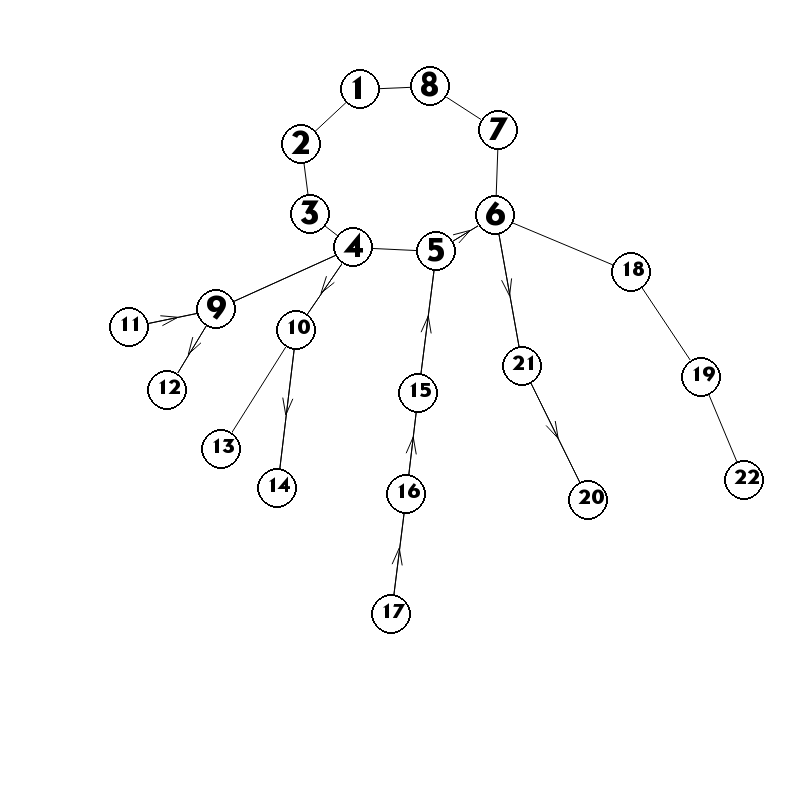## 树

$$\rm down_u = \frac{\sum_{u\rightarrow v}down_v+L_{u,v}}{son_u}\\ \rm up_u = L_{u,f}+\frac{son_fdown_f-down_u-L_{f,u}+up_f}{son_f}$$

$$\rm f_u=\begin{cases}\frac{\rm son_udown_u+up_u}{\rm son_u+1}&,\rm exist fa and son Node\\ \rm \frac{\rm son_udown_u}{\rm son_u}&,\rm exist son Node\\ \rm up_u&,\rm exist father Node \end{cases}$$

## 基环树1. 求出唯一的一个环;
2. 求出每棵树上每个点向子树中走的期望路径长度;
3. 求出每个树根向环上走的期望路径长度;
4. 求出树上每个点的向上走的期望路径长度;

### Solution

-------------本文结束感谢您的阅读-------------